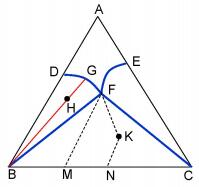## The Forms of Lever Rule in the Phase Diagram of Multi-component Systems

,,, , ,

 基金资助: 北京科技大学研究型示范教学项目MOOC课程示范项目

 Fund supported: 北京科技大学研究型示范教学项目MOOC课程示范项目Abstract

Two forms of the lever rule in the two-phase and three-phase regions of a multi-component system are discussed in this article. As for the two-component system, the cooling curves of solutions are explained with the lever rule in a three-phase line and the freezing point depression equation. A similar form of the lever rule is presented for three points on a line segment in a three-phase diagram region. This equation can be exploited to prepare the solution with desired concentrations of a multi-component system and to draw three-phase diagrams.

Keywords： Multi-component system ; Lever rule ; Phase diagram ; Phase equilibrium

WEI Mei-Ju, CHEN Fei-Wu, LI Xu-Qin, YE Ya-Ping, YUAN Wen-Xia. The Forms of Lever Rule in the Phase Diagram of Multi-component Systems. University Chemistry[J], 2016, 31(5): 73-77 doi:10.3866/PKU.DXHX201601001

## 1 两组分体系

${W_{\rm{A}}} \cdot \left|{{\rm{G}}\left. {\rm{H}} \right|} \right.{\rm{ = }}{W_{\rm{I}}} \cdot \left|{\left. {{\rm{HI}}} \right|} \right.$

### 图1DEF线是三相线，D点和F点分别对应纯A的固相和纯B的固相，E点对应熔化物液相。在三相线上是否存在杠杆原理呢？答案是肯定的，不过形式和式(1)稍有不同。设体系的物系点在C处，熔化物液相、纯固相A和B的量分别记为WEWAWB，则

$\left( {{W_{\rm{A}}} + {W_{\rm{E}}} + {W_{\rm{B}}}} \right) \cdot {x_{\rm{C}}}{\rm{ = }}{W_{\rm{E}}} \cdot {x_{\rm{E}}} + {W_{\rm{B}}}$

${W_{\rm{A}}}\left| {\left. {{\rm{DC}}} \right|} \right.{\rm{ + }}{W_{\rm{E}}}\left| {\left. {{\rm{EC}}} \right|} \right. = {W_{\rm{B}}}\left| {\left. {{\rm{CF}}} \right|} \right.$

${W_{\rm{A}}}\left| {\left. {{\rm{DE}}} \right|} \right.{\rm{ = }}{W_{\rm{B}}}\left| {\left. {{\rm{EF}}} \right|} \right.$

### 图2$\Delta {T_{\rm{f}}} = {k_{\rm{f}}}{m_{\rm{A}}}$

### 图3## 2 三组分体系

${W_{\rm{B}}}\left| {\left. {{\rm{BH}}} \right|} \right.{\rm{ = }}{W_{\rm{G}}}\left| {\left. {{\rm{HG}}} \right|} \right.$

### 图4$\left( {{W_{\rm{B}}} + {W_{\rm{C}}} + {W_{\rm{F}}}} \right) \cdot {x_{\rm{N}}}{\rm{ = }}{W_{\rm{F}}} \cdot {x_{\rm{M}}} + {W_{\rm{C}}}$

${W_{\rm{B}}}\left| {\left. {{\rm{BN}}} \right|} \right.{\rm{ + }}{W_{\rm{F}}}\left| {\left. {{\rm{MN}}} \right|} \right.{\rm{ = }}{W_{\rm{C}}}\left| {\left. {{\rm{NC}}} \right|} \right.$

### 图5$x_{\rm{A}}^{\rm{E}} = x_{\rm{A}}^{\rm{D}} + \alpha \left( {x_{\rm{A}}^{\rm{F}} - x_{\rm{A}}^{\rm{D}}} \right)$

$x_{\rm{B}}^{\rm{E}} = x_{\rm{B}}^{\rm{D}} + \alpha \left( {x_{\rm{B}}^{\rm{F}} - x_{\rm{B}}^{\rm{D}}} \right)$

$x_{\rm{C}}^{\rm{E}} = x_{\rm{C}}^{\rm{D}} + \alpha \left( {x_{\rm{C}}^{\rm{F}} - x_{\rm{C}}^{\rm{D}}} \right)$

$\beta {W_{\rm{F}}}\left| {\left. {{\rm{FE}}} \right|} \right.{\rm{ = }}{W_{\rm{D}}}\left| {\left. {{\rm{ED}}} \right|} \right.$

${W_{\rm{F}}}\left| {\left. {{\rm{FE}}} \right|} \right.{\rm{ = }}\frac{1}{\beta }{W_{\rm{D}}}\left| {\left. {{\rm{ED}}} \right|} \right.$

## 参考文献 原文顺序 文献年度倒序 文中引用次数倒序 被引期刊影响因子

Levine I.N Physical Chemistry 6th ed NewYork: McGraw-Hill, 2009.

Atkins P ; Paula J Atkins's Physical Chemistry 8th ed London: Oxford University Press, 2006.

/

 〈〉